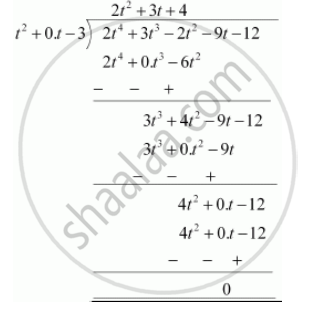Share

# Check whether the first polynomial is a factor of the second polynomial by dividing the second polynomial by the first polynomial - Mathematics

Course

#### Question

Check whether the first polynomial is a factor of the second polynomial by dividing the second polynomial by the first polynomial

t2 – 3, 2t4 + 3t3 – 2t2 – 9t – 12

#### Solution

t2 - 3, 2t4 + 3t3 - 2t2 - 9t - 12

t2 - 3 = t2 + 0.t - 3Since the remainder is 0,

Hence, t2 - 3 is a factor of 2t4 + 3t3 - 2t2 - 9t - 12

Is there an error in this question or solution?

#### APPEARS IN

NCERT Solution for Mathematics Textbook for Class 10 (2019 (Latest))
Chapter 2: Polynomials
Ex. 2.3 | Q: 2.1 | Page no. 36
RD Sharma Solution for Class 10 Maths (2018 (Latest))
Chapter 2: Polynomials
Ex. 2.3 | Q: 2.1 | Page no. 57